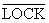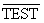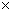Time: 3 Hours                                                                                                     Max. Marks: 100

NOTE: There are 9 Questions in all.

·      Question 1 is compulsory and carries 20 marks. Answer to Q. 1. must be written in the space provided for it in the answer book supplied and nowhere else.

·      Out of the remaining EIGHT Questions answer any FIVE Questions. Each question carries 16 marks. Questions in ITALICS are choice questions.

·      Any required data not explicitly given, may be suitably assumed and stated.

Q.1       Choose the correct or best alternative in the following:                                         (2x10)

a.       Signal voltage ranges for a logic high and for a logic low in RS-232C standard are

(A)  Low = 0 volt to 1.8 volt, high = 2.0 volt to 5 volt

(B)  Low =-15 volt to –3 vol, high = +3 volt to +15 volt

(C)    Low = +3 volt to +15 volt, high = -3 volt to -15 volt

(D)  Low = 2 volt to 5.0 volt, high = 0 volt to 1.8 volt

b.      The PCI bus is the important bus found in all the new Pentium systems because

(A)    It has plug and play characteristics

(B)    It has ability to function with a 64 bit data bus

(C)    Any Microprocessor can be interfaced to it with PCI controller or bridge

(D)    All of the above

c.   Which of the following statement is true?

(A)    The group of machine cycle is called a state.

(B)    A machine cycle consists of one or more instruction cycle.

(C)    An instruction cycle is made up of machine cycles and a machine cycle is made up of number of states.

(D)    None of the above

d.   Arrange the 8086-interrupt response in proper order.

2.    Push CS

3.    Push  IP

4.    Clear TF

5.    Clear IF

6.    Push Flags

(A)   6-5-4-3-2-1                                (B) 6-5-4-2-3-1

(C) 1-2-3-4-5-6                                 (D) 6-5-4-1-2-3

e.   In which of the following 8086 Instruction, Carry flag is not affected?

(A)     INC                                             (B)  AAS

(C)  NEG                                            (D)  None of the these

f.    Which flag of the 8086 is a control flag?

(A)     Sign Flag (SF)                              (B)  Parity Flag (PF)

(C)  Overflow Flag (OF)                     (D)  None of the above

g.   Given that

(DS) = 1200 H, (SI)=2300 H, Displacement = 1235 H, (BX)=1111 H

(A)     16646 H                                      (B)  15535 H

(C)  15850 H                                      (D)  14300 H

h.   Which of the following instruction of 8086 is invalid?

(A)    TEST AX, BX                             (B) NEG AX

(C)  MOV CX,  [AX]                         (D) None of the above

i.    8251 is a

(A)   UART

(B)   USART

(C)   Programmable Interrupt controller

(D)  Programmable interval timer/counter

j.    8088 microprocessor  has

(A) 16 bit data bus                              (B)  4 byte prefetch queue

(C)  6 byte prefetch queue                   (D) 16 bit address bus

Answer any FIVE Questions out of EIGHT Questions.

Each question carries 16 marks.

Q.2     a.   Compare 8086 and 80386 microprocessors.                                                      (4)

b.   Discuss DOS function call and BIOS function call with one example of each.                      (5)

c.  Differentiate between real and protected modes of an Intel microprocessor. Discuss protected mode memory addressing in brief.                               (7)

Q.3     a.   What is the use of the following pins on 8086?

(i)                  A19/S0-A16/S3

(ii)                QS0 and QS1

(iii)(iv)(6)

b.   Draw and discuss the timing diagram of 8086 write bus cycle.                             (6)

c.   Describe the response of 8086, when an interrupt occurs on its INTR pin.          (4)

Q.4     a.   Compare minimum mode and maximum mode of 8086.                                      (6)

b.   What do you mean by the term procedure? What is the difference between  near call  and far call.                                                             (4)

c.   Write 8086 assembly language program to add two 32-bit (i.e. four bytes) numbers. Assume that both the numbers are stored in memory locations with label Word 1 and Word 2. Store the result in next consecutive memory locations.                                                                                              (6)

Q.5     a.  Explain the following instructions of 8086 family. Explain how flags are effected when these instructions are executed.                                             (9)

(i) ENTER (ii) LEA CX, [BX] [DI] (iii) XLAT  (iv) LOCK prefix (v) SCASW  (vi) TEST

b.   Explain the following 8087 (math co-processor) instructions

(i) FSQRT (ii) FCHS (iii) FTST                                                                         (3)

c.   Why are buffers often required in an 8086 based system?                                   (2)

d.   In case of a 13 bit D/A converter, full scale output voltage is 13 Volts. Find the resolution and maximum output voltage of this converter.            (2)

Q.6     a.   Design an address decoding logic using a 3:8 decoder (74138) to interface a total of 64k memory locations in the address range from F0000 to FFFFF.  Divide 64k memory locations in eight blocks of 8 k locations each and generate eight chip select signals.                                                          (8)

b.   Draw and explain the block diagram of DMA controller. Also explain the various modes in which DMAC works. Why each channel of 8237 DMAC can at most transfer 16 K bytes of data.           (8)

Q.7     a.   What is DRAM? What do you understand by DRAM refreshing? With the help of a block diagram, show how DRAM can be interfaced to a microprocessor.                                                           (6)

b.   Discuss mode –2 (bi-directional mode) of 8255 (Programmable Peripheral Interface).                   (6)

c.   Draw and discuss the block diagram of the motherboard of a PC.                       (4)

Q.8      a.   Write the Sequence of instructions in the assembly language of 8087 to find the minimum number from a given set of numbers.                           (4)

b.   Discuss the following: (ANY THREE) (12)

(i)                 Some features of Pentium series of microprocessors.

(ii)                Virtual memory.

(iii)              MMX Technology.

(iv)              Parameter passing and recursive procedures.

Q.9     a.   Write explanatory notes on (ANY FOUR)

(i)                  Paging

(ii)                8284 Clock generator

(iii)               EISA Bus

(iv)              Assembler Directives

(v)                Hard disk drive controller

(vi)              Addressing Modes of 8086                                                                  (16)